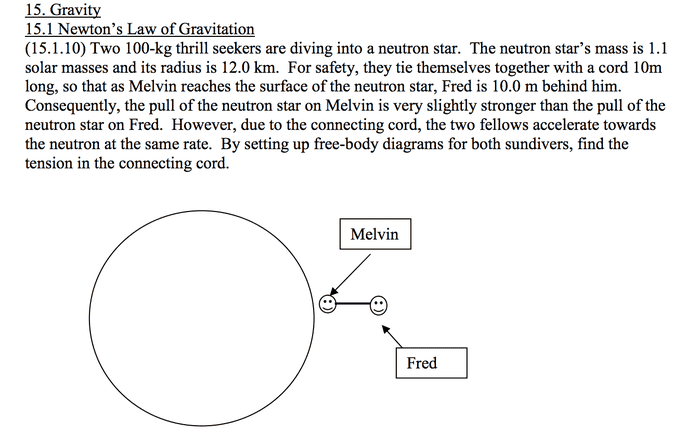# Newton's law of universal gravitation

• anirzehc

## Homework Statement

[/B]
Two 100-kg thrill seekers are diving into a neutron star. The neutron star’s mass is 1.1 solar masses and its radius is 12.0 km. For safety, they tie themselves together with a cord 10m long, so that as Melvin reaches the surface of the neutron star, Fred is 10.0 m behind him. Consequently, the pull of the neutron star on Melvin is very slightly stronger than the pull of the neutron star on Fred. However, due to the connecting cord, the two fellows accelerate towards the neutron at the same rate. By setting up free-body diagrams for both sundivers, find the tension in the connecting cord.Answer is 8.45 X 10^10 N

ΣF = ma

## The Attempt at a Solution

Mn = mass of neutron star
m1 = mass of melvin
m2= mass of fred
d = length of cord

Sum of forces on melvin
(G*Mn*m1)/ R^2 - T = m1a
Sum of forces on fred
(G*Mn*m2)/ (R+d)^2 + T = m2a

m1=m2 so
m1a = m2a

(G*Mn*m1)/ R^2 - T = (G*Mn*m2)/ (R+d)^2 + T

T = (G*Mn*m1)/ 2R^2 - (G*Mn*m2)/ 2(R+d)^2

Mn converted to kg = 2.19 x 10^30 kg
R= 1200m
G= 6.67 x 10^-11
d = 10m
m1=m2=100kg

Plugging everything in I am getting 8.35 x 10^13 N but the answer is 8.45x 10^10 N, what am i doing wrong

Your method and final formula for T look correct. Check the final calculation where you plug in the numbers.
Note that the radius is 12 km, which is not equal to 1200 m.

omg i just noticed 12 km is 12000 m not 1200 thanks